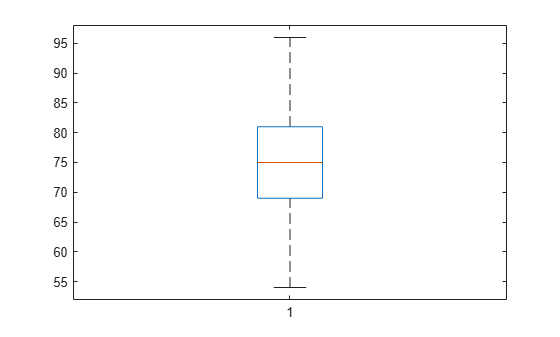# iqr

Interquartile range of probability distribution

## Syntax

``r = iqr(pd)``

## Description

example

````r = iqr(pd)` returns the interquartile range of the probability distribution `pd`. The scalar value of `r` is the difference between the values of the 75th and 25th percentiles of the probability distribution.```

## Examples

collapse all

Create a standard normal distribution object with the mean, $\mu$, equal to 0 and the standard deviation, $\sigma$, equal to 1.

`pd = makedist('Normal','mu',0,'sigma',1);`

Compute the interquartile range of the standard normal distribution.

`r = iqr(pd)`
```r = 1.3490 ```

The returned value is the difference between the 75th and the 25th percentile values for the distribution. This is equivalent to computing the difference between the inverse cumulative distribution function (icdf) values at the probabilities y equal to 0.75 and 0.25.

`r2 = icdf(pd,0.75) - icdf(pd,0.25)`
```r2 = 1.3490 ```

Load the sample data. Create a vector containing the first column of students’ exam grade data.

```load examgrades; x = grades(:,1);```

Create a normal distribution object by fitting it to the data.

`pd = fitdist(x,'Normal')`
```pd = NormalDistribution Normal distribution mu = 75.0083 [73.4321, 76.5846] sigma = 8.7202 [7.7391, 9.98843] ```

Compute the interquartile range of the fitted distribution.

`r = iqr(pd)`
```r = 11.7634 ```

The returned result indicates that the difference between the 75th and 25th percentile of the students’ grades is 11.7634.

Use `icdf` to determine the 75th and 25th percentiles of the students’ grades.

`y = icdf(pd,[0.25,0.75])`
```y = 1×2 69.1266 80.8900 ```

Calculate the difference between the 75th and 25th percentiles. This yields the same result as `iqr`.

`y(2)-y(1)`
```ans = 11.7634 ```

Use `boxplot` to visualize the interquartile range.

`boxplot(x)`The top line of the box shows the 75th percentile, and the bottom line shows the 25th percentile. The center line shows the median, which is the 50th percentile.

## Input Arguments

collapse all

Probability distribution, specified as one of the probability distribution objects in the following table.

Distribution ObjectFunction or App Used to Create Probability Distribution Object
`BetaDistribution``makedist`, `fitdist`, Distribution Fitter
`BinomialDistribution``makedist`, `fitdist`, Distribution Fitter
`BirnbaumSaundersDistribution``makedist`, `fitdist`, Distribution Fitter
`BurrDistribution``makedist`, `fitdist`, Distribution Fitter
`ExponentialDistribution``makedist`, `fitdist`, Distribution Fitter
`ExtremeValueDistribution``makedist`, `fitdist`, Distribution Fitter
`GammaDistribution``makedist`, `fitdist`, Distribution Fitter
`GeneralizedExtremeValueDistribution``makedist`, `fitdist`, Distribution Fitter
`GeneralizedParetoDistribution``makedist`, `fitdist`, Distribution Fitter
`HalfNormalDistribution``makedist`, `fitdist`, Distribution Fitter
`InverseGaussianDistribution``makedist`, `fitdist`, Distribution Fitter
`KernelDistribution``fitdist`, Distribution Fitter
`LogisticDistribution``makedist`, `fitdist`, Distribution Fitter
`LoglogisticDistribution``makedist`, `fitdist`, Distribution Fitter
`LognormalDistribution``makedist`, `fitdist`, Distribution Fitter
`LoguniformDistribution``makedist`
`MultinomialDistribution``makedist`
`NakagamiDistribution``makedist`, `fitdist`, Distribution Fitter
`NegativeBinomialDistribution``makedist`, `fitdist`, Distribution Fitter
`NormalDistribution``makedist`, `fitdist`, Distribution Fitter
`PiecewiseLinearDistribution``makedist`
`PoissonDistribution``makedist`, `fitdist`, Distribution Fitter
`RayleighDistribution``makedist`, `fitdist`, Distribution Fitter
`RicianDistribution``makedist`, `fitdist`, Distribution Fitter
`StableDistribution``makedist`, `fitdist`, Distribution Fitter
`tLocationScaleDistribution``makedist`, `fitdist`, Distribution Fitter
`TriangularDistribution``makedist`
`UniformDistribution``makedist`
`WeibullDistribution``makedist`, `fitdist`, Distribution Fitter

## Version History

Introduced in R2013a